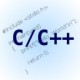# Let’s Make a Deal

I originally discussed this problem in the old Singularity blog as a result of it having been mentioned on a Numb3rs episode. Numerous people asked me if the show made a mistake. I’ve been around enough to recognize the old “Let’s Make a Deal” problem from the famous old game show of the same name.

When the problem was first posed to me (a very long time ago), I made the correct choice but only because I took the ‘intuitive’ answer and chose the opposite. My first analysis of the problem (done in the early 90’s along with a Fortran simulator) is reposted below. Shortly after the first Singularity post, a Flash simulator was provided at the lessrain blog.

A recent conversation about the non-intuitive nature of many results from Statistics lead me to druge this up. Perhaps I’ll do a Flex simulator some time in the future.

Original Singularity post follows

——

Here is the modern version of “Let’s Make a Deal”. You are a contestant on a game show. The host offers you a choice of three doors.  Behind one of the doors is a lifetime pass to Flash In the Can. Behind the other two doors is a rotten apple or some equally undesirable item.  You receive whatever is behind the door you select.

Suppose you select door #1.  The host opens door #3 to reveal one of the rotten apples.  The host gives you an option to either stick with door #1 or switch to door #2.

Would you be better off to switch, stay, or does it not make any difference?  Conventional wisdom states that since only two choices remain and both are equally likely, the probability that either door contains the prize is 1/2. So, switching or staying makes no difference.  In reality, your chances of winning the prize double by switching.  The solution to the problem is non-intuitive and sparked a great deal of debate when the solution was proposed in a column by Marilyn vos Savant.  The intuitive approach treats the problem as one of absolute probability, when it is actually an exercise in conditional probability.

A simple (but non-rigorous) approach to the correct solution breaks the problem into two sets.  S is the singleton consisting of your selected door. S’ is the set of the two doors you did not select.  If P(S) is the probability that the prize is behind the door you selected, then P(S) = 1/3. P(S’) = 2/3.  When the host reveals what is behind one of the doors in S’, neither P(S) or P(S’) changes.  The host revealed nothing about S, but did reveal something about S’.  By switching rom S to S’, you double the probability of selecting the door containing the prize.  For a somewhat more rigorous analysis, consider the game conditions

1: Each door is equally likely to contain the prize.
2: There is only one prize. The other two doors hide something undesirable.
3: The host knows in advance which door contains the prize and *always* opens a door containing the non-desirable item before offering the choice to switch or stay.

The location of the prize door and contestant’s selection make no difference to the analysis, so for simplicity, let’s stick with the original contestant selection of door #1.

Define the events

H1: Host opens door #1
H2: Host opens door #2
H3: Host opens door #3

P1 Prize behind door #1
P2 Prize behind door #2
P3 Prize behind door #3

Recall that P(A|B) is the probability of event A given that event B occurred. Note that P(H1|any event) = 0 and P(P1) = P(P2) = P(P3) = 1/3 . For events A and B, P(A and B) = P(A)P(B|A).

From the initial conditions, P(H2|P1) = 1/2, P(H2|P2) = 0, P(H2|P3) = 1, P(H3|P1) = 1/2, P(H3|P2) = 1, P(H3|P3) = 0

The table of intersections is below. The ij-th entry in the table is the probability of Pi and Hj. For example, P(P1 and H2) = P(P1)P(H2|P1).

```    H1   H2         H3
P1  0   (1/3)*(1/2) (1/3)*(1/2)

P2  0   (1/3)*0     (1/3)*1

P3  0   (1/3)*1     (1/3)*0```

As a check, the row sums should each be 1/3. For example, P(P1) = P(H1 and P1) + P(H2 and P1) + P(H3 and P1) or all the ways the prize could be behind door #1. The column sums are the probability the host opened a particular door.

You select door #1. The host opens door #3 to reveal the rotten apple. You are given the option to stay with door #1 or switch to door #2. To determine which choice is better, compare the probability that the prize is behind #1 given that the host opens #3 to the probability that the prize is behind #2 given that the host opens #3. P(P1|H3) = P(H3 and P1)/P(H3) P(P2|H3) = P(H3 and P2)/P(H3). Using the intersection values from the table,

P(P1|H3) = ‘stay’ = (1/6)/(1/3 + 1/6) = (1/6)/(3/6) = 1/3

P(P2|H3) = ‘switch’ = (1/3)/(1/3 + 1/6) = (1/3)/(3/6) = (1/3)/(1/2) = 2/3.

—–

This is a very old problem that has been discussed numerous times online, so google “Monty Hall” or “Let’s Make a Deal” for more information. Also, check out that lessrain simulator and be glad that Flash development does not require statistics 🙂

## 4 thoughts on “Let’s Make a Deal”

1.Keith Peters says:

Classic. I did a Flash sim of this a few years ago too. 🙂

2.algorithmist says:

Keith – that’s cool. Do you have a link to share as well?

3.edgarsr says:

Amazing!

4.drawk says:

Interesting stuff indeed! Thanks for reviving it, I have seen this before but after delving into game theory in the last 2-3 years it is entirely a different perspective. Stuff like this is as intriguing as quantum viewing changes, where it just doesn’t seem right until you model it.

From a famous line from Princess Bride, “inconztheezvable”.